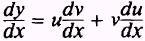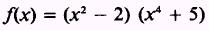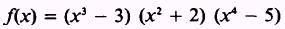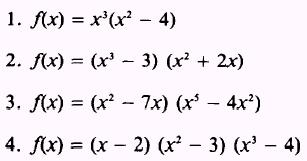Custom SearchPRODUCTS Theorem 4. The derivative of the product of two differentiable functions of x is equal to the first function multiplied by the derivative of the second function, plus the second function multiplied by the derivative of the first function. IfthenThis theorem may be extended to include the product of three differentiable functions or more. The result for three functions would be as follows: ifthenEXAMPLE. Find the derivative ofSOLUTION. The derivative of the first factor is 2x, and the derivative of the second factor is 4x3. Therefore,EXAMPLE. Find the derivative ofSOLUTION. The derivatives of the three factors, in the order given, are 3x2, 2x, and 4x3. Therefore,Expanding, we getPRACTICE PROBLEMS: Find the derivatives of the following:ANSWERS:Integrated Publishing, Inc. - A (SDVOSB) Service Disabled Veteran Owned Small Business